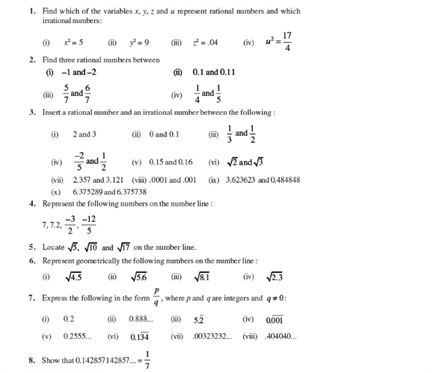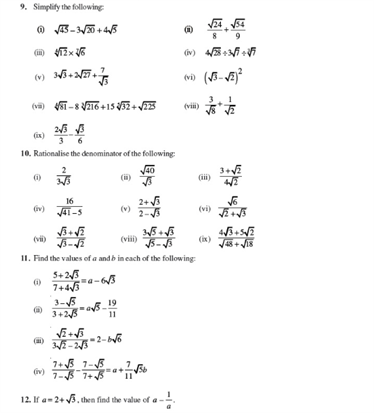# Revision Notes For CBSE Class 9 Math Chapter -1 Number Systems

A number system is a method mainly used to represent or express numbers. It is a collection of different symbols which are called digits. The different types of number systems are:

• Binary number system (Base- 2)
• Octal number system (Base-8)
• Decimal number system (Base- 10)
• Hexadecimal number system (Base- 16)

The most commonly used number system is the decimal number system which is also called as the Hindu-Arabic numeral system and it was developed over 1000 years ago.There are different types of numbers in the number system. They include natural numbers, whole numbers, even number, an odd number, real numbers, integers, rational numbers, etc. Apart from these, there are even more ways to classify numbers and this was the most important and common classifications of numbers.

## CBSE Class 9 Math revision notes for Chapter 1- Number Systems